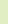The best free certification study guides, practice tests and forums!Join Us! | Login | HelpCertifications Microsoft CompTIA Cisco CIW LPI Red Hat IBM
 Forum Stats Fatal error: Call to a member function sql_error() on a non-object in /host/www/forums/includes/functions.php on line 558
 Links TechTutorials CertifyPro Certnotes A+ Tutorials# Exam Hints: Subnetting

By Orion IV

On the 70-291 exam, there is a good chance you will be tested on how well you understand IP addressing and different scenarios where IP subnetting is used.

First, you must have a thorough understanding of how an IP address is built from binary and octets, the default A, B, C, D and E class networks and what a subnet mask and default gateway are. Second, this paper will guide you through some of the more advanced topics and tricky questions you might see in one form or another at the test.

We have 3 subnets  A, B and C. We have a host called Workstation 1. We need to add more subnets (D and E) and we have been given a class C network address 192.168.6.0 to use for this.

So lets look at what kind of trouble or problems you might be presented with.

Example 1:
You may be asked which subnet a host belongs to given its IP address or given that two hosts are on the same network what would their subnet mask be?

If we look at the figure, we have a lot of 192.168.5.x networks (subnet A, B and C), so which one does the host Workstation 1 belong to? Since we have the subnet masks given for each subnet we must figure out what ranges the individual subnets cover to identify the range where 128 fits in. We could use a binary cheat-sheet, but lets look at the bytes to be thorough. First we take each host from the different subnets and convert to binary by looking only at the last octet:

Host 140 = 10001100
Host 130 = 10000010
Subnet 240 = 11110000
Host 35 = 00100011
Host 62 = 00111110
Host 22 = 00010110
Subnet 192 = 11000000
Host 66 = 01000010
Host 128 = 10000000

So with all the hosts lined up we can see that the subnets are identified as the 1000 network, the 00 network and the 01 network given the subnet masks for each subnet. The 128 host only matches the 1000 network because its 4 first bits mismatch even the first 2 bits of the 11 and 01 networks. The 192.168.5.128 should be given the subnet mask of 255.255.255.240 and be placed on Subnet A.

We can use the decimal to binary conversion to compare two hosts and determine what subnet mask they should have to be on the same network  the opposite of the above method:

Host A = 11011011
Host B = 11101101

So if we line up the binary numbers for the hosts, we can see that they differ on the 3rd bit in this octet. Given the previous octets are alike, the network bits stop at the 2nd and the host bits start from the 3rd. This would give them subnet mask 192 for that octet.

Remember, these kinds of questions might be camouflaged with other information to set you off such as:
• A DNS server where Round Robin has not been enabled for multiple Web Servers hosting the same material  which Web Server will a host connect to?
• Wrongfully configured IP addresses on hosts, routers with incorrect subnet masks, or a misplaced host: Host A (IP/mask) = 192.168.1.92 / 255.255.255.192 is placed on the subnet/mask = 192.168.1.0/ 255.255.255.128. This subnet already covers the hosts 192.168.1.1 to 192.168.1.126. Given the subnet mask 255.255.255.192, the host will only be able to communicate with hosts in the range of 192.168.1.65 to 192.168.1.126 as it will believe the first 62 hosts are on a different subnet.
Example 2:
Occasionally, you might be presented with a question about how to divide a single network into X number of networks and figure out how many hosts will be supported? Or, if there are Y number of hosts, then how many networks? Often this will be subnetting with different length subnet masks.

Given the 192.168.6.0 class C network, how can we divide this into subnets of 100 hosts and 30 hosts respectively? The train of thought here is to divide in halves and then divide the second half in more but smaller parts.

If we divide the network into to parts, there will be 126 hosts on each, so we have more than 100 for the first subnet. We then assign Subnet D the range 192.168.6.0/25 (192.168.6.1 to 168.192.6.126 valid for hosts with subnet mask 266.255.255.128).

Then we divide the remaining portion of the hosts (128-254) into 2 or more subnets. Since we only need 30 hosts we could either make some subnets with 62 hosts or more with 32 hosts.

• So the next subnet Subnet E would be either 192.168.6.128/26 with 62 hosts or 192.168.6.128/27 with only 30 hosts but with no room to grow. The answer depends on the question and what solutions we can choose from when taking the test.

Enjoy!

•Sponsors